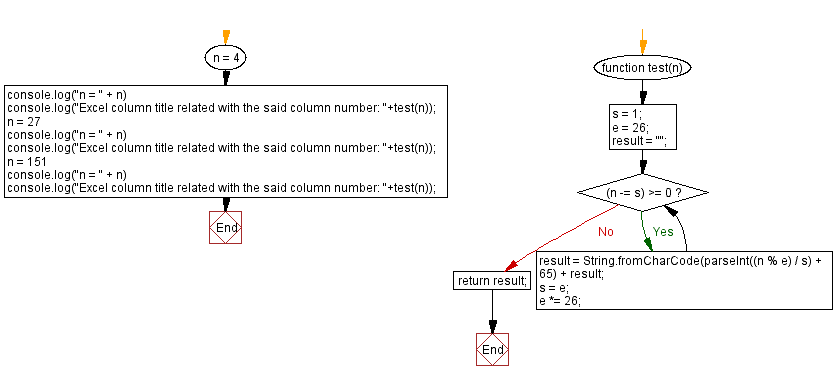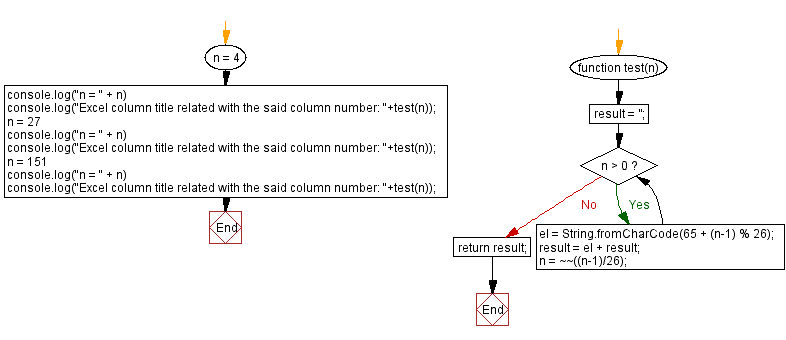# JavaScript: Excel column title related with a column number

## JavaScript Math: Exercise-97 with Solution

Write a JavaScript program to retrieve the Excel column title associated with a given column number (integer value).

For example:
1 -> A
2 -> B
3 -> C
...
26 -> Z
27 -> AA
28 -> AB
...

Visualisation:Test Data:
(3) -> C
(27) -> AA
( 151) -> EU

Sample Solution-1:

HTML Code:

``````<!DOCTYPE html>
<html>
<meta charset="utf-8">
<title>JavaScript program to Excel column title related with a column number</title>
<body>

</body>
</html>
```
```

JavaScript Code:

``````function test(n) {
s = 1;
e = 26;
result = "";
while ((n -= s) >= 0) {
result = String.fromCharCode(parseInt((n % e) / s) + 65) + result;
s = e;
e *= 26;
}
return result;
}
n = 4
console.log("n = " + n)
console.log("Excel column title related with the said column number: "+test(n));
n = 27
console.log("n = " + n)
console.log("Excel column title related with the said column number: "+test(n));
n = 151
console.log("n = " + n)
console.log("Excel column title related with the said column number: "+test(n));
```
```

Sample Output:

```n = 4
Excel column title related with the said column number: D
n = 27
Excel column title related with the said column number: AA
n = 151
Excel column title related with the said column number: EU
```

Flowchart:Live Demo:

See the Pen javascript-math-exercise-97 by w3resource (@w3resource) on CodePen.

Sample Solution-2:

JavaScript Code:

``````function test(n) {
result = '';
while(n > 0){
el = String.fromCharCode(65 + (n-1) % 26);
result = el + result;
n = ~~((n-1)/26);
}
return result;
}
n = 4
console.log("n = " + n)
console.log("Excel column title related with the said column number: "+test(n));
n = 27
console.log("n = " + n)
console.log("Excel column title related with the said column number: "+test(n));
n = 151
console.log("n = " + n)
console.log("Excel column title related with the said column number: "+test(n));
```
```

Sample Output:

```n = 4
Excel column title related with the said column number: D
n = 27
Excel column title related with the said column number: AA
n = 151
Excel column title related with the said column number: EU
```

Flowchart:Live Demo:

See the Pen javascript-math-exercise-97-1 by w3resource (@w3resource) on CodePen.

Improve this sample solution and post your code through Disqus

What is the difficulty level of this exercise?

Test your Programming skills with w3resource's quiz.

﻿